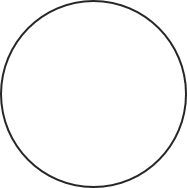# Circle Area Calculator

This is an online circle area calculator that helps you find the area of a circle. The circle area formula will be explained. Examples of circle area calculations are provided, too.

## What is a circle?

A circle is a simple closed shape consisting of all points in a plane that are a given distance from a given center point. This distance from the center to any point on the circle is called the radius.## Circle area formula

The area of a circle is calculated as follows:

`area = πr2`

where r is the radius of the circle

## How to find the area of a circle?

You can use the circle area calculator to find the area of a circle:

1. Enter the length of the radius of the circle.
2. Our circle calculator performs all the calculations for you. The diameter, circumference, and area are displayed.

#### This calculator is created with

the best online spreadsheet editor with excellent formula and editing capability.

For more calculators for finance, mathematics health, unit converters and more check out our calculators collection.